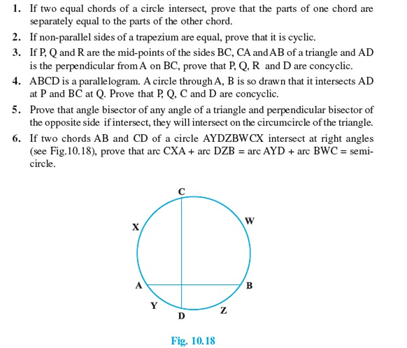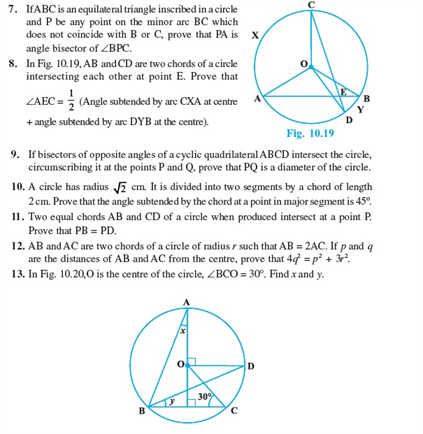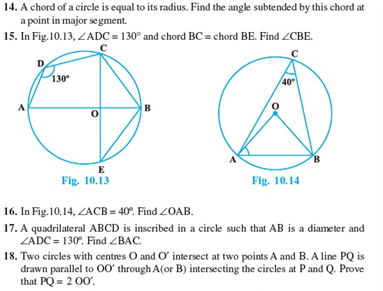# Revision Notes For CBSE Class 9 Math Chapter -10 Circles

In geometry, a circle is defined as a closed figure with a set of points in a plane at a specific distance from the centre. It is divided into two parts: an interior and an exterior.

The area of a circle is calculated by the given formulae: A = π r2

Where: A is the Area and r is the radius.

The properties of circle are:

• The line passing through the centre of a circle is called a Diameter.
• The distance around the circle is called as the Circumference.
• The distance from the centre to the circumferenceof a circle is called as the Radius.

Check here for the free CBSE Class 9 Math revision notes for Chapter 10- Circles